# Domain

Jump to: navigation, search

A non-empty connected open set in a topological space. The closure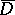of a domainis called a closed domain; the closed set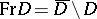is called the boundary of. The points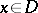are also called the interior points of; the pointsare called the boundary points of; the points of the complement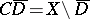are called the exterior points of.

Any two points of a domainin the real Euclidean space,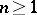(or in the complex space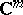,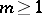, or on a Riemann surface or in a Riemannian domain), can be joined by a path (or arc) lying completely in; ifor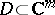, they can even be joined by a polygonal path with a finite number of edges. Finite and infinite open intervals are the only domains in the real line; their boundaries consist of at most two points. A domainin the plane is called simply connected if any closed path incan be continuously deformed to a point, remaining throughout in. In general, the boundary of a simply-connected domain in the (open) plane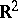or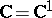can consist of any numberof connected components,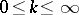. Ifis regarded as a domain in the compact extended plane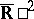or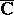and the numberof boundary components is finite, thenis called the connectivity order of; for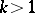,is called multiply connected. In other words, the connectivity orderis one more than the minimum number of cross-cuts joining components of the boundary in pairs that are necessary to makesimply connected. For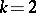,is called doubly connected, for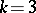, triply connected, etc.; for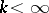one has finitely-connected domains and for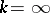infinitely-connected domains. The connectivity order of a plane domain characterizes its topological type. The topological types of domains in,, or in,, cannot be characterized by a single number.

Even for a simply-connected plane domainthe metric structure of the boundarycan be very complicated (see Limit elements). In particular, the boundary points can be divided into accessible points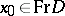, for which there exists a path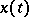,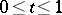,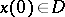,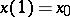, joininginwith any point, and inaccessible points, for which no such paths exists (cf. Attainable boundary point). For any simply-connected plane domainthe set of accessible points of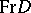is everywhere dense in.

A domaininoris called bounded, or finite, ifif not,is called unbounded or infinite. A closed plane Jordan curve divides the planeorinto two Jordan domains: A finite domain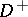and an infinite domain. All boundary points of a Jordan domain are accessible.

#### Comments

Instead of, the boundary ofis also denoted by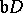or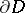.

From the definition it can be seen that a domain is bounded if (and only if) it is contained in a ball centred at the coordinate origin and of finite radius.

How to Cite This Entry:
Domain. E.D. Solomentsev (originator), Encyclopedia of Mathematics. URL: http://www.encyclopediaofmath.org/index.php?title=Domain&oldid=17271
This text originally appeared in Encyclopedia of Mathematics - ISBN 1402006098